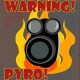## Making particles follow a path

So I was tasked to make a particle system follow a set of points (P1, P2, P3, … Pn) for a visualization in the CAVE. Basically given a particle system, make the particles in it follow a set of points:If we linearly interpolate their positions through a group of points we will get a set of particles that will follow a set of points, something like this:Instead what we want is to make each spawned particle follow a set of directions, something like this:So in order to achieve this, we create an array of directions (given the points), then we check the total distance traveled for each particle and then depending on where the particle is located (across the points) we change the direction accordingly towards the next point (when it reaches each point)

The end result:

### The Code:

The code is pretty straight forward; one editor script and one simple script that needs to be attached to a game object that contains the particle system.

##### The script:

Still there is some stuff missing like adding speed to the particles or making the generated pivots move relative to the game object but it pretty much does the job.

### One Response to “Making particles follow a path”

1.Tim32 says:

Good!